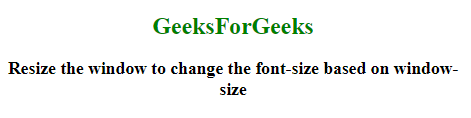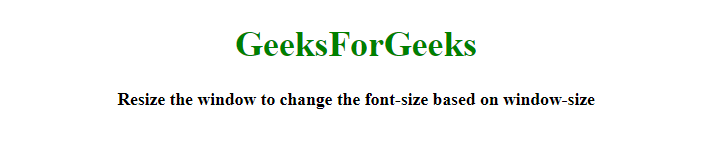# How to set font size based on window size using JavaScript ?

Given an HTML document and the task is to change the font-size based on the size of the window with the help of JavaScript.

Approach 1:

• First convert the document element font sizes to em or % by using a function.
• Call this function on every resize of the window. It will change the font size based on window size.

Example: This example implements the above approach.

 `  ` `<``html``>  ` ` `  `<``head``>  ` `    ``<``title``>  ` `        ``How to set font size based on ` `        ``container size using JavaScript? ` `    `` ` ` ` ` `  `<``body` `style` `= ``"text-align:center;"``>  ` `     `  `    ``<``h1` `id` `= ``"h1"` `style` `= ``"color: green"``>  ` `        ``GeeksForGeeks  ` `    `` ` `     `  `    ``<``p` `id` `= ``"GFG_UP"` `style` `= ` `        ``"font-weight: bold;"``> ` `    `` ` `     `  `    ``<``script``>  ` `        ``var up = document.getElementById('GFG_UP'); ` `        ``var h1 = document.getElementById('h1'); ` `         `  `        ``up.innerHTML = "Resize the window to change" ` `            ``+ "the font-size based on window-size"; ` `         `  `        ``h1.setFont = function (font) { ` `            ``var size = this.offsetWidth, ` `            ``font_size = size * font; ` `            ``this.style.fontSize = font_size + '%'; ` `            ``return this ` `        ``}; ` `         `  `        ``h1.setFont(0.50); ` `         `  `        ``window.onresize = function () { ` `            ``h1.setFont(0.50); ` `        ``} ` `    ``  ` `  ` ` `  ` `

Output:

• Before resizing the window:• After resizing the window:Approach 2: Use vw(viewport) unit with the font-size to convert the font-size with respect to the viewport.

Example: This example implements the above approach.

 `  ` `<``html``>  ` ` `  `<``head``>  ` `    ``<``title``>  ` `        ``How to set font size based on ` `        ``container size using JavaScript? ` `    `` ` `     `  `    ``<``style``> ` `        ``#h1 { ` `            ``font-size: 5vw; ` `        ``} ` `    `` ` ` ` ` `  `<``body` `style` `= ``"text-align:center;"``>  ` `     `  `    ``<``h1` `id` `= ``"h1"` `style` `= ``"color: green"``>  ` `        ``GeeksForGeeks  ` `    `` ` `     `  `    ``<``p` `id` `= ``"GFG_UP"` `style` `= ` `        ``"font-weight: bold;"``> ` `    `` ` `         `  `    ``<``script``>  ` `        ``var up = document.getElementById('GFG_UP'); ` `        ``var h1 = document.getElementById('h1'); ` `        ``up.innerHTML = "Resize the window to change" ` `             ``+ "the font-size based on window-size"; ` `    ``  ` `  ` ` `  ` `

Output:

• Before resizing the window:• After resizing the window:My Personal Notes arrow_drop_upCheck out this Author's contributed articles.

If you like GeeksforGeeks and would like to contribute, you can also write an article using contribute.geeksforgeeks.org or mail your article to contribute@geeksforgeeks.org. See your article appearing on the GeeksforGeeks main page and help other Geeks.

Please Improve this article if you find anything incorrect by clicking on the "Improve Article" button below.